# Test: Spectroscopy Level - 1

## 35 Questions MCQ Test Organic Chemistry | Test: Spectroscopy Level - 1

Description
Attempt Test: Spectroscopy Level - 1 | 35 questions in 70 minutes | Mock test for Chemistry preparation | Free important questions MCQ to study Organic Chemistry for Chemistry Exam | Download free PDF with solutions
QUESTION: 1

### A molecule, AX, has a vibrational energy of 1000 cm–1 and rotational energy of 10 cm–1. Another molecule, BX, has a vibrational energy of 400 cm–1 and rotational energy of 40 cm–1. Which one of the following statements about the coupling of vibrational and rotational motion is true:

Solution:

More the rotational energy, more will be the coupling.

Therefore, The coupling is stronger in BX.

QUESTION: 2

Solution:
QUESTION: 3

### In the IR spectrum, carbonyl absorption band for the following compound appears at: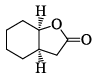Solution:
QUESTION: 4

In the IR spectrum of p-nitrophenyl acetate, the carbonyl absorption band appears at:

Solution:
QUESTION: 5

A triatomic molecule of the type AB2 shows two IR absorption lines and one IR-Raman line. The structure of the molecule is:

Solution:

B cannot be as its both IR - Raman active, and according to the rule of mutual exclusion, a molecule having centre of symmetry only one of them can be active.

QUESTION: 6

In the IR spectrum, the absorption band due to carbonyl group in phenyl acetate appears at:

Solution:
QUESTION: 7

In the IR spectrum of p-nitrophenyl, acetate, the carbonyl absorption band appears at:

Solution:
QUESTION: 8

A compound with molecule formula C4H6O2 shows band at 1170 cm–1 in IR spectrum and peaks at 178, 68, 28 and 22 ppm in 13C NMR spectrum. The correct structure of the compound is:

Solution:
QUESTION: 9

The compound that exhibits sharp bands at 3300 and 2150 cm–1 in the IR spectrum is:

Solution:
QUESTION: 10

Correctly matched structure and carbonyl stretching frequency set is: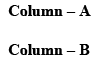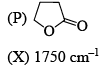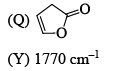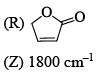Solution:
QUESTION: 11

Among the following, the compound that displays an IR band at 2150 cm–1 is:

Solution:
QUESTION: 12

The compound which shows IR frequencies at both 3314 and 2126 cm–1 is:

Solution:
QUESTION: 13

For the molecule CBr4, the number of peaks which comprise the cluster for the molecular ion will be which of the following?

Solution:

Since bromine has two separate isotopes (79 and 81, with approximately the same proportions of each), the possibilities for different molecular masses will be those with four 79 isotopes, three 79 and one 81 isotope, two of each isotope, three 81s and one 79 isotopes, and four 81 isotopes - i.e. 5 in total.

QUESTION: 14

The IR stretching frequencies (cm–1) for the compound X are as follows: 3300-3500 (s, br); 3000 (m); 2225 (s); 1680(s). The correct assignment of the absorption bands is: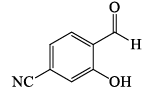Solution:
QUESTION: 15

Among the compounds given in the option (a) to (d), the one that exhibits a sharp band at around 3300 cm–1 in the IR spectrum is:

Solution:
QUESTION: 16

The correct order of IR stretching frequency of the C=C in the following olefins is: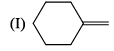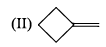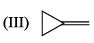Solution:
QUESTION: 17

Arrange the chacacteristic timescales of the following set of dynamical spectroscopic techniques in decreasing order of time (longest to shortest); NMR (Nuclear Magnetic Resonance), ESR (Electron Spin Resonance), Fluorescence, Raman and Absorption.

Solution:
QUESTION: 18

What are the limits of detection of the following common analytical methods used with capillary separations: fluorescence, mass spectrometry, UV-Vis absorbance, and NMR, respectively, in mol:

Solution:
QUESTION: 19

Of the molecules CH4, CO2, benzene and H2, the ones that will absorb infrared radiation are:

Solution:
QUESTION: 20

IR stretching frequencies of carbonyl groups in aldehydes and acid chlorides in cm–1 are:

Solution:
QUESTION: 21

The bond that gives the most intense band in the infrared spectrum for its stretching vibrations is:

Solution:

Approximate Infrared Stretching Frequencies:

• C–H - 3000 cm-1
• N–H - 3600 cm-1
• O–H - 3600 cm-1
• S–H - 2570 cm-1
QUESTION: 22

An examinations of saturated hydrocarbons containing methyl group show asymmetrical (Vas) and symmetrical (Vs) stretching modes in the region of:

Solution:
QUESTION: 23

For each set of compounds below, choose the one in which the indicated hydrogen is farthest upfield in a proton NMR spectrum: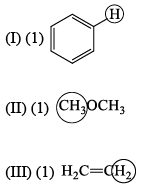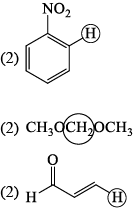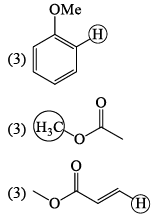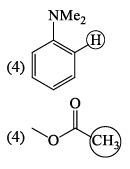Solution:

In NMR spectroscopy upfield means the shielded protons. Hence the farthest upfield are the ones that are most effectively shielded from NMR spectroscopy rays.

According to the above discussion A is correct.

QUESTION: 24

The molecule with the smallest rotational constant (in the microwave spectrum) among the following is:

Solution:
QUESTION: 25

N2 does not show pure vibrational spectral because:

Solution:
• A homonuclear diatomic molecule like H2, O2, N2, etc. which have only stretching motion/vibrations and no bending motion/vibrations, the dipole moment does not change during vibration.
• Hence these molecules do not give vibration spectra i.e. they are said to be infrared-inactive.
QUESTION: 26

Which of the following molecules has the lowest vibrational stretching frequency:

Solution:

C is correct because more the reduced mass, less the vibrational stretching frequency.

QUESTION: 27

A radiation that has an energy of N 50 kJ mol–1 falls in the following region of the electromagnetic spectrum:

Solution:
• The electromagnetic spectrum illustrates all the possible electromagnetic radiations, their categorization on the basis of energies, frequencies, wavelengths.
• In the electromagnetic spectrum, energy is expressed in electron volt.
∴ First, we convert kJ to eV
i.e. 1 Joule = 6.242 × 1018 eV
1eV = (1.60217733 × 10^-22) × (6.0223 × 10^23) = 96.49 kJ/mole
⇒ 50 kJ/mole = 0.485 eV
• This eV falls in the infrared radiation.
QUESTION: 28

The rotational constant (B) of H35Cl, H37Cl and D35Cl follow the order:

Solution:

The value of B decreases with isotopic substitution.

QUESTION: 29

The spectroscopic data for an organic compound with molecular formula C10H12O2 are given below. IR band around 1750 cm–1. 1H NMR d 7.3 (m, 5H), 5.85 (q, 1H, J = 7.2 Hz), 2.05 (s, 3H), 1.5 (d, 3H, J = 7.2 Hz) ppm. The compound is:

Solution:
QUESTION: 30

The correct order of the 1H NMR chemical shift values (β) for the indicated hydrogens (in bold) in the following compounds is: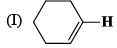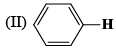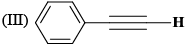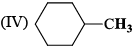Solution:
QUESTION: 31

The correct order of 1H NMR chemical shift (δ) values for the labeled methyl groups in the following compound is: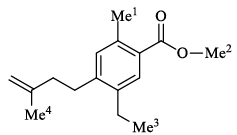Solution: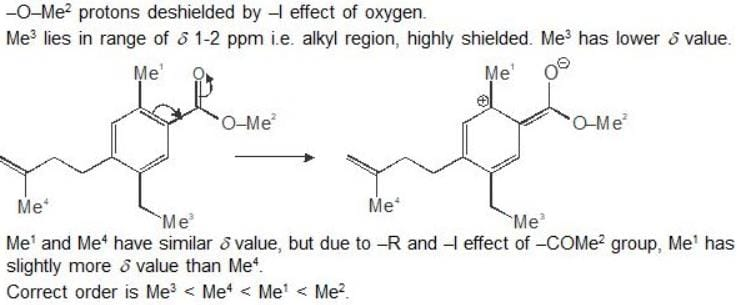QUESTION: 32

A C5H12O2 compound has strong infrared absorption at 3300 to 3400cm-1. The 1H NMR spectrum has three singlets at β 0.9, β 3.45 and β 3.2 ppm with relative areas 3:2:1. Addition of D2O to the sample eliminates the lower field signal. The 13C NMR spectrum shows three signals all at higher field than β 100 ppm. Which of the following compounds best fits this data:

Solution:
QUESTION: 33

A compound of formula C5H12 gives one signal in the 1H NMR and two signals in the 13C NMR spectra. The compound is:

Solution:
QUESTION: 34

The most appropriate spectroscopy for the identification of a nitrile group is:

Solution:
QUESTION: 35

Compound I gives a strong infrared absorption at 1730 cm-1. 1H NMR spectrum indicates that it has two types of hydrogen atoms; one H atom appearing as signlet at δ = 9.7 ppm and 9H atoms appearing as a singlet at δ = 1.2 ppm. The structure of I is:

Solution:Use Code STAYHOME200 and get INR 200 additional OFF Use Coupon Code